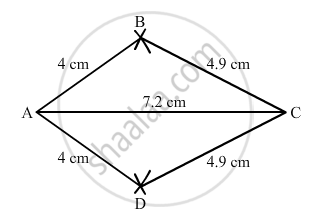Share

# Construct a Kite Abcd in Which Ab = 4 Cm, Bc = 4.9 Cm and Ac = 7.2 Cm. - Mathematics

Course
ConceptConstructing a Quadrilateral When Two Diagonals and Three Sides Are Given

#### Question

Construct a kite ABCD in which AB = 4 cm, BC = 4.9 cm and AC = 7.2 cm.

#### Solution

$\text { Steps of construction }:$

$\text { Step I: Draw AC = 7 . 2 cm } .$

$\text { Step II: With A as the centre and radius 4cm, draw arcs on both sides of the line segment AC }.$

$\text { Step III : With C as the centre and radius 4 . 9 cm, draw arcs on both sides of AC intersecting the previous arcs of step II at B and D }.$

$\text { Step IV: Join BA, DA, BC and CD .}$

$\text { Thus, the quadrilateral ABCD so obtained is the required kite } .$Is there an error in this question or solution?

#### APPEARS IN

RD Sharma Solution for Mathematics for Class 8 by R D Sharma (2019-2020 Session) (2017 to Current)
Chapter 18: Practical Geometry (Constructions)
Ex. 18.1 | Q: 6 | Page no. 4
Solution Construct a Kite Abcd in Which Ab = 4 Cm, Bc = 4.9 Cm and Ac = 7.2 Cm. Concept: Constructing a Quadrilateral - When Two Diagonals and Three Sides Are Given.
S